# Understanding Q-learning: How a Reward Is All You Need

There are two general ways to train an AI to match a given expectation: we can either give it the expected outputs (commonly named labels) for differents inputs; we call this supervised learning. Or we can provide a reward for each output as a score: this is reinforcement learning (RL).

Supervised learning works by tweaking all the parameters (weights in neural networks) to fit the desired outputs, expecting that given enough input/label pairs the AI will find common rules that generalize for any input.

Reinforcement learning’s reward is often provided from a simple function that can score any output: we don’t know what specific output would be best, but we can recognize how good the result is. In this latter statement there are two underlying concepts we will address in this post:

• Can we only tell if the output is good in a binary way, or do we have to quantify the output to train our AI?
• Do we have to give a reward for every AI’s output? Can we give a reward only at specific times?

Those questions are already mostly answered, and many algorithms deal with those topics. Our journey here will be to understand how we tackle those questions and end up with a beautiful formula that is at the core of modern approaches of RL:Equation 1. Q estimation at the heart of many RL algorithm, also known as the Bellman equation.

## Q-learning

The vast majority, if not all, of modern RL algorithms are based on the principles of Q-learning: the idea is to evaluate a ‘reward expectation’ for each possible action. If we can have a good evaluation, we could maximize the reward by choosing actions with the maximum evaluated rewards. The function giving this expected reward is named Q. For now, we will assume we can have a reward for any action.Equation 2. Definition of the Q function.

The `t` indices show that the state and action aren’t constant and will vary, usually with time/action taken. On the other hand, the `Q` function and the reward function `r` are unique functions that ideally return the ’expected reward’ for any (state, action) pairs.

For now, we will assume we can have a reward that gives an objective and perfect evaluation of each state/action.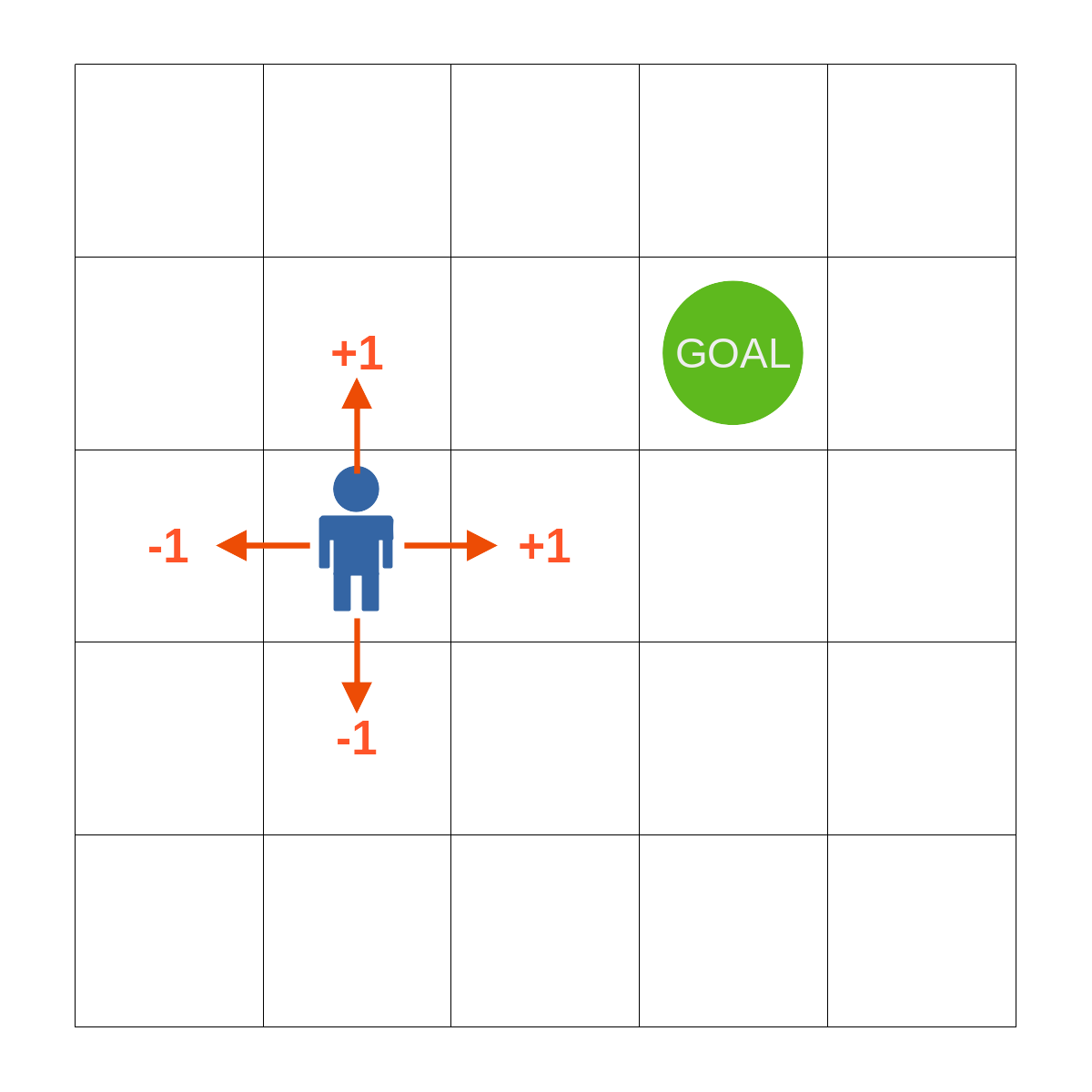Figure 1. Example of reward given for different actions at a specific state. Here a simple 2D map with a goal.

### Q-Table

We know that actions’ outcomes (rewards) will vary depending on the current state we are in, otherwise the problem would be trivial to solve. If the states that are relevant to our actions can be numbered, a simple way would be to build a table with all the possible states/action pairs. There are different ways to build such a table depending on how we can interact with our environment. Eventually, we would have a good ‘map’ to guide us to do the best actions.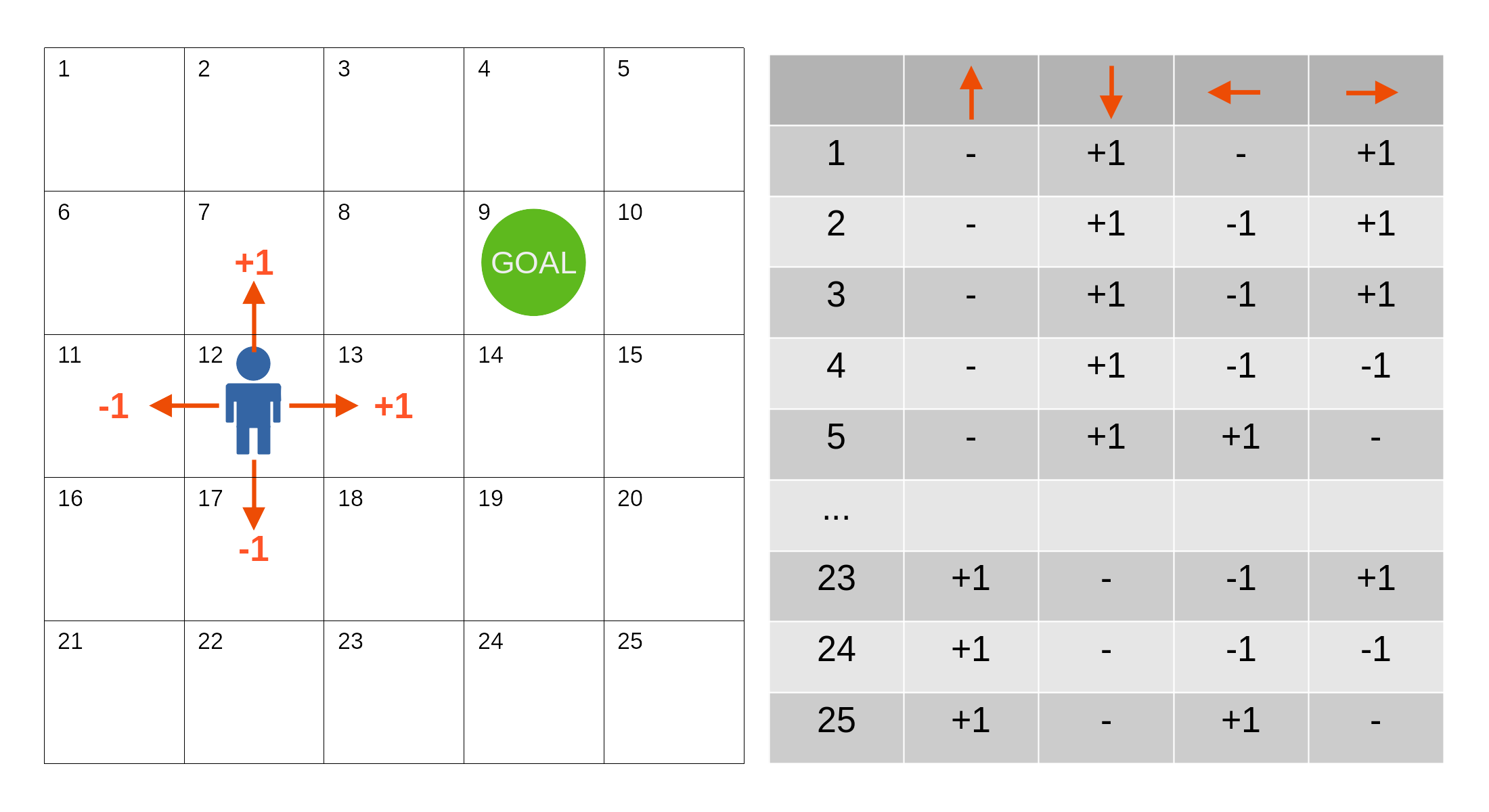Figure 2. Example of Q-table: we can build an exhaustive table for all the possible (state, action) pairs

### Deep Q-Learning

When the number of variables of the environment relevant to our actions/rewards becomes too large, the number of possible states grows quickly. It doesn’t take a lot of possible parameters to make the Q-table approach unfeasible. Neural networks are known to work very nicely and efficiently in high dimensionality (with many input variables). They also generalize well, so the idea in Deep Q-Learning is to use a neural network to predict the different Q values for each action given a state.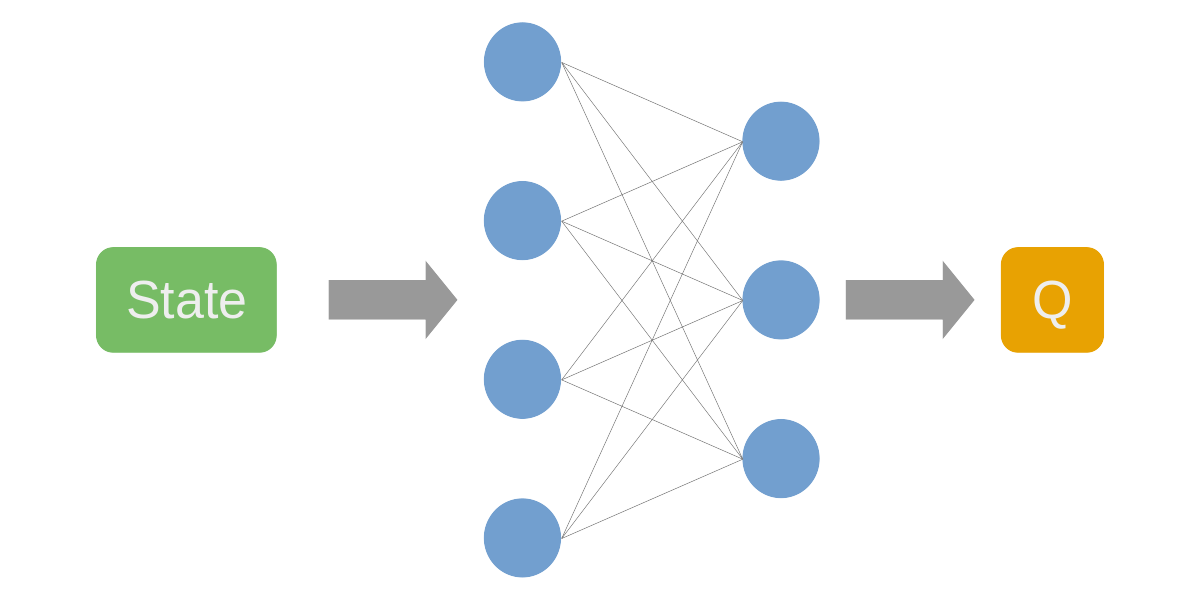Figure 3. A neural network can predict Q values from state information

In this case, we do not need to give the state/action pairs but only the state, as the neural network would exhaustively return all the Q values associated with each action. Outputting all actions’ Q value is a common method as the general cases have a complex environment but a smaller number of possible actions.

This method works very well. It is similar to supervised learning with states as inputs and rewards as labels. We assumed so far that we had a reward for each action, and we chose the next action with the best reward (called a greedy policy). In many cases this is not enough: even if an action would yield the best reward at a given state, this may affect the next state so that we wouldn’t optimize the reward in the long term. Also, if we can’t have a reward for each action, we usually give 0 as a reward. We will not be able to choose the right action if they affect later states despite not yielding different rewards at the current state.

The sparsity of rewards or the long-term calculation of total reward (non-greedy policies) leads us to diverge from supervised learning and learn potential future rewards.

## Temporal difference: TD-Learning

TD-learning is a clever way to account for potential future value without knowing them yet. TD is a model-free class of algorithms: it does not simulate future states. The main idea is to consider all the rewards of a sequence of actions to give a better value than just the reward of the next action.

We can, for instance, sum all the future rewards: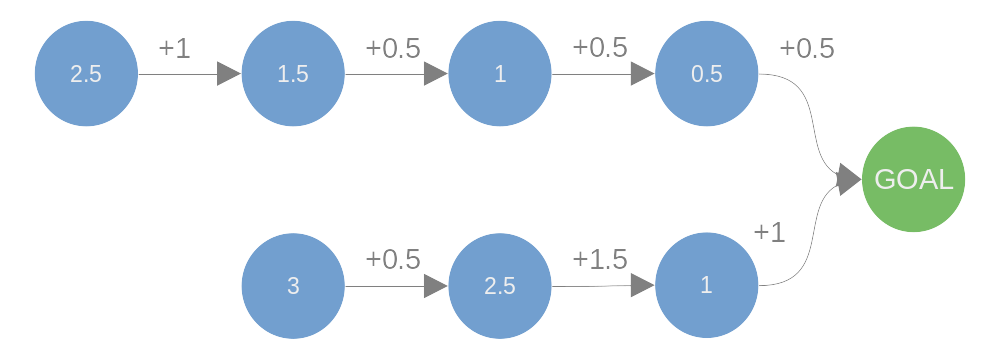Figure 4. Cumulating future rewards to assign values to each state.

Mathematically this can be written as:Equation 3.

This is named TD(0): the simplest form of TD method, accumulating all the rewards.

### Introducing policies

We could try different trajectories (sequence of actions) and retrospectively get the final reward for each action, but this has 2 drawbacks: the environment is usually too vast, and the sequence of actions might not even have a definite end. Also, such exhaustive methods might not be very efficient. Instead, we can evaluate the ‘value’ of the next state overall, like the maximum of all its possible rewards (direct reward), and add this value to the reward of a given action.

If a state can have different branches, we can select the best one, and this would be our policy, the way we choose actions. This simple form of taking the maximum is called the ‘greedy’ policy.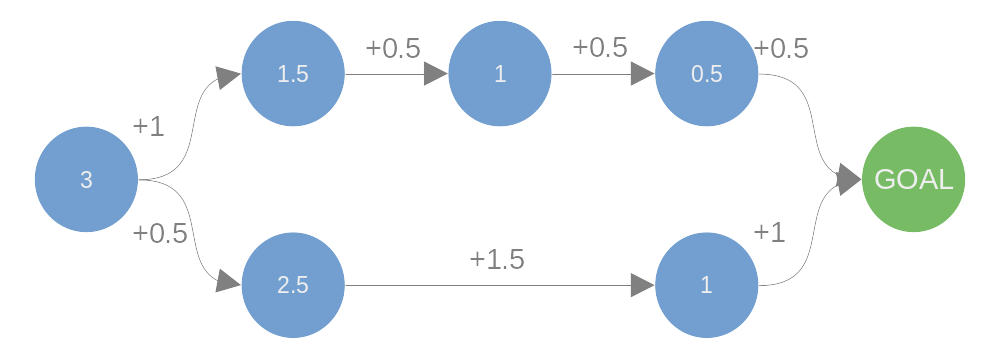Figure 5. With a greedy policy the associated values to state come from the maximum value of the next state. Here despite the lower branch giving only half the top reward directly the overall value is greater.

This can be written down as:Equation 4.

The expected value notation is defined as:Equation 5.

For a greedy policy the probabilities `p` would all be set to 0 but the one associated with the highest return to 1 (in case of equality between n actions, we would attribute ‘1/n’ as probabilities to get the same expected value).Equation 6.

### Relation with Q function

The expected reward can be replaced by the Q function we used earlier, which now can be denominated to be specific to our chosen policy (named π):Equation 7.

### TD-0

We previously discussed the problem of not being able to go through all the states exhaustively and that the evaluation of the Q value from a neural network could help. We want to use the TD method to have a better value estimation that will consider potential future rewards.

The TD(0) method is elegant as we can, in fact, only use the next state’s expected value instead of all future ones. The idea is that with successive evaluations, we build a chain of dependencies as each states’ value depends on the next one.Equation 8.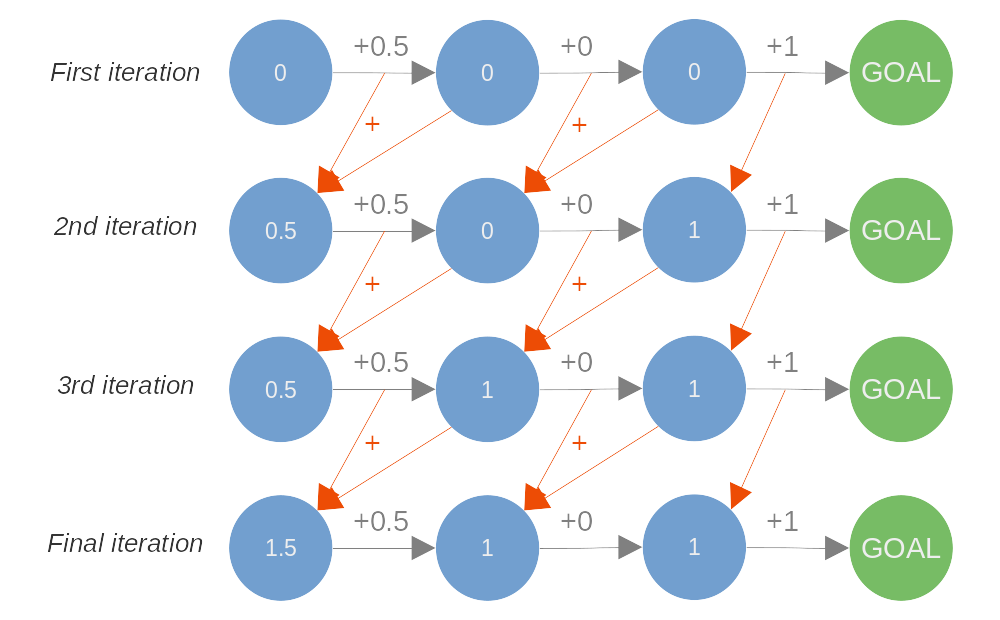Figure 6. Iterative propagation of state values following TD(0) method.

We can see that the greedy policy would work even with null rewards in the trajectory. We can explicit our greedy policy, going back to use Q value instead of the state value V:Equation 9.

### TD-lambda

We need to fix a problem: if a trajectory grows too long or never ends, a state value can potentially grow indefinitely. To counter that, we can add a discount factor (originally named lambda, usually refer as gamma in Q-learning) for the next state’s value:Equation 10.

Notice that we simplify the reward notation for clarity.

To avoid exploding values, this discount has to be between 0 and 1 (strictly below 1). We can think about it as giving more importance to the direct reward than the future ones. As the contribution to the latter reward decrease, the chain of action can grow without the calculated value growing. If the reward has an upper limit, the value will also be bounded.

The sparsity of rewards is also solved: giving only a positive reward after many non-rewarding steps will create smooth values for the intermediate states. Any reward, positive or negative, will diffuse its value to the neighbor states.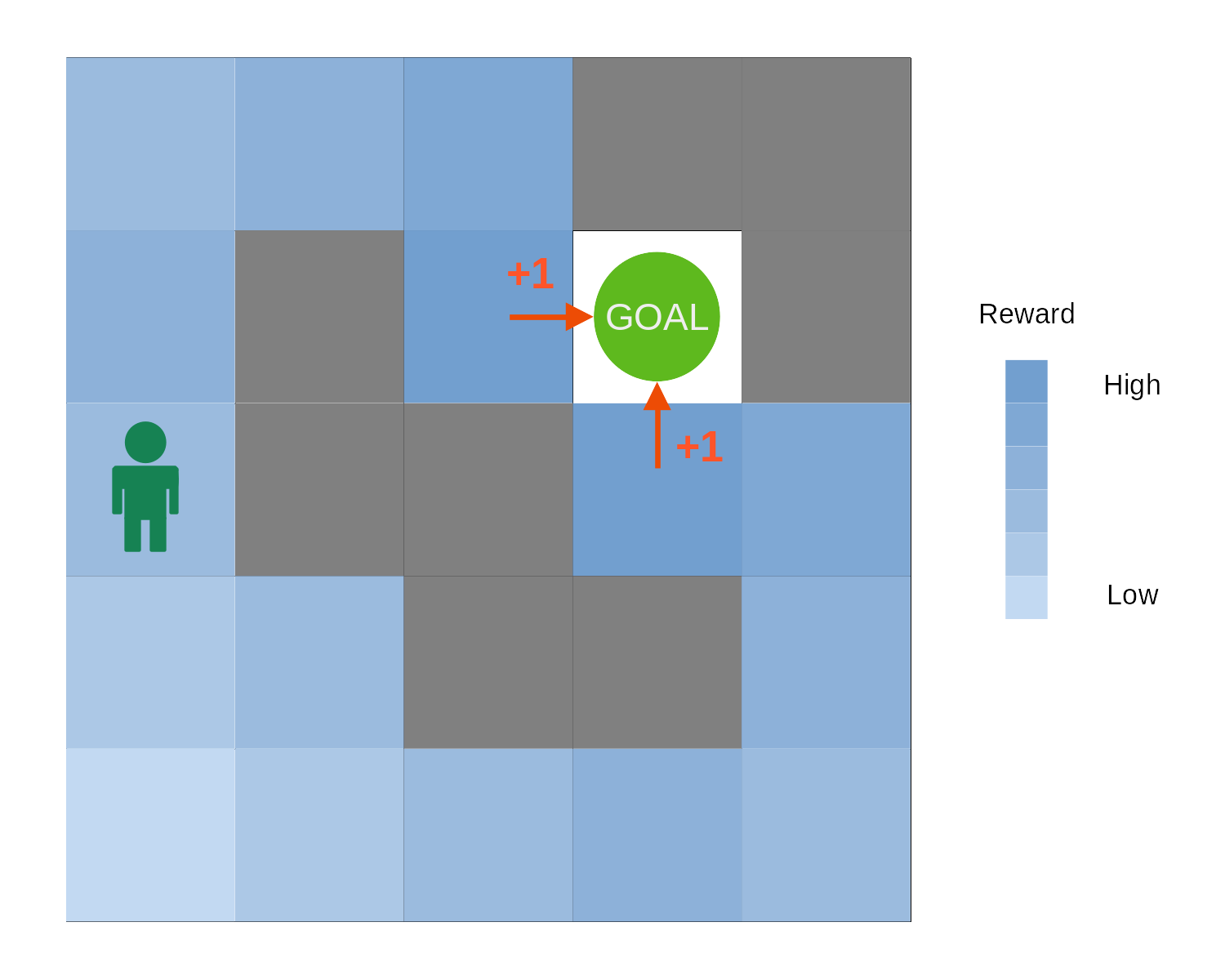Figure 7. The TD(0) value propagation can allow for a smooth value distribution over the state that will help building efficient behaviour.

## Q-Learning algorithm

Finally, as we train a neural network to estimate the Q function, we need to update its target with successive iteration. We cannot fully trust the estimator (a neural network here) to give the correct value, so we introduce a learning rate to update the target smoothly.Equation 11. Fully explained Bellman equation.

That is it! We now understand all the parts of this formula. Over multiple training steps with different sates, the training should find a good average Q function. While training, the estimator uses its own output to train itself (commonly referred to as bootstrapping): it is like it is chasing itself. Bootstrapping can lead to instability in the training process. There are many additional methods to help against such instability.

From giving rewards, sparse or not, binary or fine-grained, we have a smooth space of values for all our states/actions so the AI can follow a greedy policy to the best outcome.

This way of training is not a silver bullet and there is no guarantee that the AI will find a correlation from the information given as state to the returned reward.

## Conclusion

We can see how our rewards are used to train AI’s policies using Q-learning. By understanding the many iterations required and the bootstrapping issues, we can help our AI by carefully giving relevant state information and reward:

• There needs to be a correlation between the state information and the reward: the simpler the relationship, the easier/faster the AI will find it.
• Sparse and binary rewards make the training problem long and arduous. Giving more information through the reward can tremendously increase the speed/accuracy of the learned Q-estimator.
• The longer the chain of actions, the more complex the Q-value will be to estimate.

We didn’t see how the AI’s algorithm can explore different actions given an environment here. Spice.ai’s technology focuses exclusively on off-policy training where we only have past data and cannot interact with the environment. RL is a vast topic and currently quickly growing. Robotics is a fantastic field of application; many other areas are yet to be explored with such a technology. We hope to push forward the technology and its field of application with our platform.

If you’d like to partner with us on the mission of making new applications by leveraging RL, we invite you to discuss with us on Discord, reach out on Twitter or email us.

I hope you enjoy this post and learn new things.

Corentin